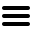vocabulary
1000+ books

# assume used in And Then There Were None

2 meanings, 4 uses
(click/touch triangles for details)
1  —3 uses as in:
I assume it's true
Definition
to accept something as true without proof
• Lombard said: "I suppose that's why we assumed it must be a stranger moving about up here."
p. 124.6
assumed = accepted as true (without really knowing)
Other Uses (with this meaning)
• Mr. Justice Wargrave said: "You are assuming heresay to be evidence."
p. 140.5
• assuming = accepting as true (without proof)
• In all this, I have assumed that the mystery of Indian Island will remain unsolved.
p. 274.1
assumed = accepted as true (without really knowing)
There are no more uses of "assume" flagged with this meaning in And Then There Were None.

Typical Usage  (best examples)
2  —1 use as in:
She assumed power
Definition
beginning to take power or responsibility
• Now he assumed command with the ease born of a long habit of authority.
p. 134.5
assumed = took on (power or responsibility)
There are no more uses of "assume" flagged with this meaning in And Then There Were None.

Typical Usage  (best examples)